Search

Conics: Hyperbolas: Finding Information
From the Equation
(page 2 of 3)

Sections: Introduction, Finding information from the equation, Finding the equation from information• Find the center, vertices, foci, eccentricity, and asymptotes of the hyperbola with the given equation, and sketch: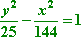• Since the y part of the equation is added, then the center, foci, and vertices will be above and below the center (on a line paralleling the y-axis), rather than side by side.

Looking at the denominators, I see that a2 = 25 and b2 = 144, so a = 5 and b = 12. The equation c2  a2 = b2 tells me that c2 = 144 + 25 = 169, so c = 13, and the eccentricity is e = 13/5. Since x2 = (x  0)2 and y2 = (y  0)2, then the center is at (h, k) = (0, 0). The vertices and foci are above and below the center, so the foci are at (0, 13) and (0, 13), and the vertices are at (0, 5) and (0, 5).

 Because the y part of the equation is dominant (being added, not subtracted), then the slope of the asymptotes has the a on top, so the slopes will be m = ± 5/12. To graph, I start with the center, and draw the asymptotes through it, using dashed lines: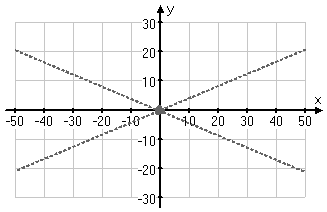Then I draw in the vertices, and rough in the graph, rotating the paper as necessary and "eye-balling" for smoothness: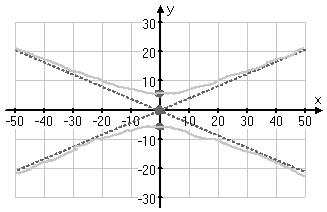Then I draw in the final graph as a neat, smooth, heavier line: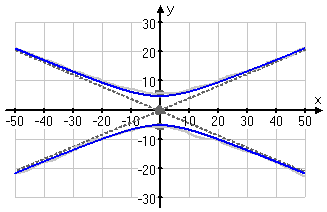And the rest of my answer is:

center (0, 0), vertices (0, 5) and (0, 5), foci (0, 13) and (0, 13),
eccentricity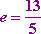, and asymptotes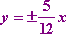• Give the center, vertices, foci, and asymptotes for the hyperbola
with equation: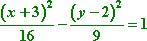• Since the x part is added, then a2 = 16 and b2 = 9, so a = 4 and b = 3. Also, this hyperbola's foci and vertices are to the left and right of the center, on a horizontal line paralleling the x-axis.

From the equation, clearly the center is at (h, k) = (3, 2). Since the vertices are a = 4 units to either side, then they are at (7, 2) and at (1, 2). The equation c2  a2 = b2 gives me c2 = 9 + 16 = 25, so c = 5, and the foci, being 5 units to either side of the center, must be at (8, 2) and (2, 2). Copyright © Elizabeth Stapel 2010-2011 All Rights Reserved

Since the a2 went with the x part of the equation, then a is in the denominator of the slopes of the asymptotes, giving me m = ± 3/4. Keeping in mind that the asymptotes go through the center of the hyperbola, the asymptes are then given by the straight-line equations  y  2 = ± (3/4)(x + 3).

center (3, 2), vertices (7, 2) and (1, 2), foci (8, 2) and (2, 2),
and asymptotes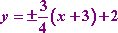• Find the center, vertices, and asymptotes of the hyperbola with equation
4x2  5y2 + 40x  30y  45 = 0.
• To find the information I need, I'll first have to convert this equation to "conics" form by completing the square.

4x2 + 40x  5y2  30y = 45
4(x2 + 10x        )  5(y2 + 6y       ) = 45 + 4(   )  5(  )

4(x2 + 10x + 25)  5(y2 + 6y + 9) = 45 + 4(25)  5(9)

4(x + 5)2  5(y + 3)2 = 45 + 100  45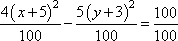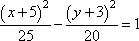Then the center is at (h, k) = (5, 3). Since the x part of the equation is added, then the center, foci, and vertices lie on a horizontal line paralleling the x-axis; a2 = 25 and b2 = 20, so a = 5 and b = 2sqrt. The equation a2 + b2 = c2 gives me c2 = 25 + 20 = 45, so c = sqrt = 3sqrt. The slopes of the two asymptotes will be m = ± (2/5)sqrt. Then my complete answer is:

center (5, 3), vertices (10, 3) and (0, 3),

foci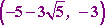and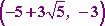,

and asymptotes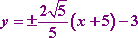If I had needed to graph this hyperbola, I'd have used a decimal approximation of ± 0.89442719... for the slope, but would have rounded the value to something reasonable like m = ± 0.9.

<< Previous  Top  |  1 | 2 | 3  |  Return to Index  Next >>

 Cite this article as: Stapel, Elizabeth. "Conics: Hyperbolas: Finding Information From the Equation." Purplemath.     Available from https://www.purplemath.com/modules/hyperbola2.htm.     Accessed [Date] [Month] 2016

Study Skills Survey

Tutoring from Purplemath
Find a local math tutor